# JS 项目中究竟应该使用 Object 还是 Map？| 项目复盘

5,899

## 前言

• 用法的区别：在某些情况下的用法会截然不同
• 句法的区别：创建以及增删查改的句法区别
• 性能的区别：速度和内存占用情况

## 用法对比

1. 对于 `Object` 而言，它键（key）的类型只能是字符串，数字或者 `Symbol`；而对于 `Map` 而言，它可以是任何类型。（包括 Date，Map，或者自定义对象）

2. `Map` 中的元素会保持其插入时的顺序；而 `Object` 则不会完全保持插入时的顺序，而是根据如下规则进行排序:

• 非负整数会最先被列出，排序是从小到大的数字顺序
• 然后所有字符串，负整数，浮点数会被列出，顺序是根据插入的顺序
• 最后才会列出 `Symbol``Symbol` 也是根据插入的顺序进行排序的
3. 读取 `Map` 的长度很简单，只需要调用其 `.size()` 方法即可；而读取 `Object` 的长度则需要额外的计算： `Object.keys(obj).length`

4. Map 是可迭代对象，所以其中的键值对是可以通过 `for of` 循环或 `.forEach()` 方法来迭代的；而普通的对象键值对则默认是不可迭代的，只能通过 `for in` 循环来访问（或者使用 `Object.keys(o)、Object.values(o)、Object.entries(o)` 来取得表示键或值的数字）迭代时的顺序就是上面提到的顺序。

``````const o = {};
const m = new Map();
o[Symbol.iterator] !== undefined; // false
m[Symbol.iterator] !== undefined; // true
``````
5. `Map` 中新增键时，不会覆盖其原型上的键；而在 `Object` 中新增键时，则有可能覆盖其原型上的键:

``````Object.prototype.x = 1;
const o = {x:2};
const m = new Map([[x,2]]);
o.x; // 2，x = 1 被覆盖了
m.x; // 1，x = 1 不会被覆盖
``````
6. `JSON` 默认支持 `Object` 而不支持 `Map`。若想要通过 `JSON` 传输 `Map` 则需要使用到 `.toJSON()` 方法，然后在 `JSON.parse()` 中传入复原函数来将其复原。

对于 `JSON` 这里就不具体展开了，有兴趣的朋友可以看一下这：JSON 的序列化和解析

``````const o = {x:1};
const m = new Map([['x', 1]]);
const o2 = JSON.parse(JSON.stringify(o)); // {x:1}
const m2 = JSON.parse(JSON.stringify(m)) // {}
``````

## 句法对比

### 创建时的区别

Obejct

``````const o = {}; // 对象字面量
const o = new Object(); // 调用构造函数
const o = Object.create(null); // 调用静态方法 Object.create
``````

Map

``````const m = new Map(); // 调用构造函数
``````

`Object` 不同，`Map` 没有那么多花里胡哨的创建方法，通常只会使用其构造函数来创建。

### 新增/读取/删除元素时的区别

Obejct

``````const o = {};
//新增/修改
o.x = 1;
o['y'] = 2;
//读取
o.x; // 1
o['y']; // 2
//或者使用 ES2020 新增的条件属性访问表达式来读取
o?.x; // 1
o?.['y']; // 2
//删除
delete o.b;
``````

• 属性名不能包含空格和标点符号
• 属性名不能以数字开头

Map

``````const m = new Map();
//新增/修改
m.set('x', 1);
//读取
map.get('x');
//删除
map.delete('b');
``````

``````const m = new Map([['x',1]]);
// 若想要将 x 的值在原有基础上加一，我们需要这么做：
m.set('x', m.get('x') + 1);
m.get('x'); // 2

const o = {x: 1};
// 在对象上修改则会简单许多：
o.x++;
o.x // 2
``````

## 性能对比

### 创建时的性能

``````let n,  n2 = 5;
// 速度
while (n2--) {
let p1 = performance.now();
n = 10000;
while (n--) { let o = {}; }
let p2 = performance.now();
n = 10000;
while (n--) { let m = new Map(); }
let p3 = performance.now();
console.log(`Object: \${(p2 - p1).toFixed(3)}ms, Map: \${(p3 - p2).toFixed(3)}ms`);
}
// 内存
class Test {}
let test = new Test();
test.o = o;
test.m = m;
``````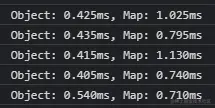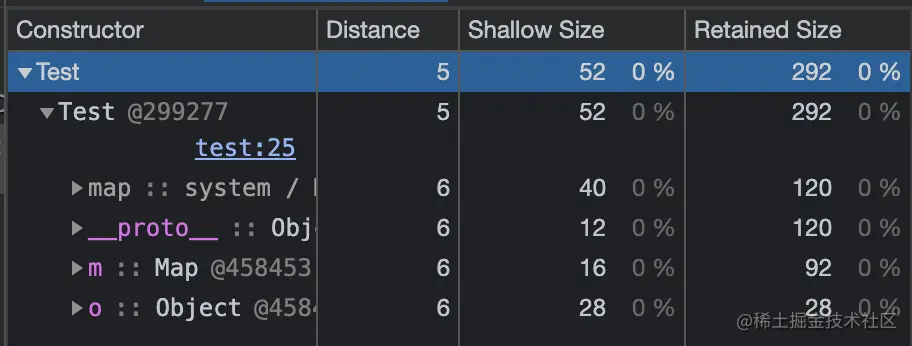### 新增元素时的性能

``````console.clear();
let n,  n2 = 5;
let o = {}, m = new Map();
// 速度
while (n2--) {
let p1 = performance.now();
n = 10000;
while (n--) { o[Math.random()] = Math.random(); }
let p2 = performance.now();
n = 10000;
while (n--) { m.set(Math.random(), Math.random()); }
let p3 = performance.now();
console.log(`Object: \${(p2 - p1).toFixed(3)}ms, Map: \${(p3 - p2).toFixed(3)}ms`);
}
// 内存
class Test {}
let test = new Test();
test.o = o;
test.m = m;
``````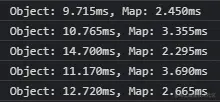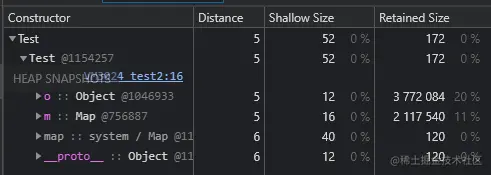### 读取元素时的性能

``````let n;
let o = {}, m = new Map();

n = 10000;
while (n--) { o[Math.random()] = Math.random(); }
n = 10000;
while (n--) { m.set(Math.random(), Math.random()); }

let p1 = performance.now();
for (key in o) { let k = o[key]; }
let p2 = performance.now();
for ([key] of m) { let k = m.get(key); }
let p3 = performance.now();
`Object: \${(p2 - p1).toFixed(3)}ms, Map: \${(p3 - p2).toFixed(3)}ms`
``````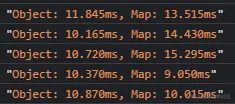### 删除元素时的性能

``````let n;
let o = {}, m = new Map();

n = 10000;
while (n--) { o[Math.random()] = Math.random(); }
n = 10000;
while (n--) { m.set(Math.random(), Math.random()); }

let p1 = performance.now();
for (key in o) { delete o[key]; }
let p2 = performance.now();
for ([key] of m) { m.delete(key); }
let p3 = performance.now();
`Object: \${(p2 - p1).toFixed(3)}ms, Map: \${(p3 - p2).toFixed(3)}ms`
``````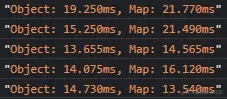### 特殊情况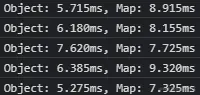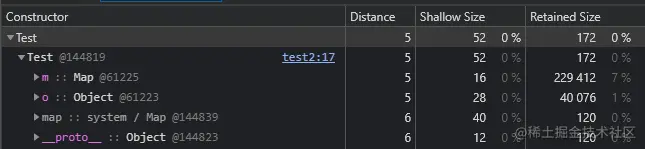## 总结

• 储存的键不是字符串/数字/或者 `Symbol` 时，选择 `Map`，因为 `Object` 并不支持
• 储存大量的数据时，选择 `Map`，因为它占用的内存更小
• 需要进行许多新增/删除元素的操作时，选择 `Map`，因为速度更快
• 需要保持插入时的顺序的话，选择 `Map`，因为 `Object` 会改变排序
• 需要迭代/遍历的话，选择 `Map`，因为它默认是可迭代对象，迭代更为便捷

• 只是简单的数据结构时，选择 `Object`，因为它在数据少的时候占用内存更少，且新建时更为高效
• 需要用到 `JSON` 进行文件传输时，选择 `Object`，因为 `JSON` 不默认支持 `Map`
• 需要对多个键值进行运算时，选择 `Object`，因为句法更为简洁
• 需要覆盖原型上的键时，选择 `Object`

### 参考

Fast properties in V8

Shallow, Retained, and Deep Size

Slow delete of object properties in JS in V8

ES6 — Map vs Object — What and when?

JavaScript高级程序设计（第4版）

JavaScript: The Definitive Guide (7th Edition)